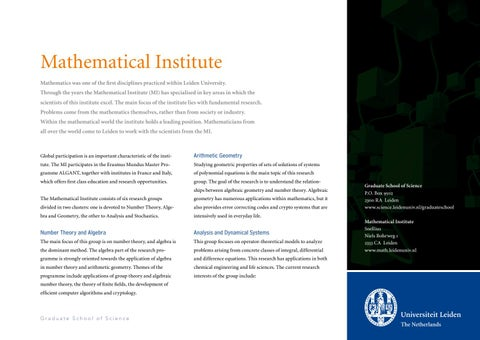Mathematical Institute Mathematics was one of the first disciplines practiced within Leiden University. Through the years the Mathematical Institute (MI) has specialised in key areas in which the scientists of this institute excel. The main focus of the institute lies with fundamental research. Problems come from the mathematics themselves, rather than from society or industry. Within the mathematical world the institute holds a leading position. Mathematicians from all over the world come to Leiden to work with the scientists from the MI.

Global participation is an important characteristic of the insti-

Arithmetic Geometry

tute. The MI participates in the Erasmus Mundus Master Pro-

Studying geometric properties of sets of solutions of systems

gramme ALGANT, together with institutes in France and Italy,

of polynomial equations is the main topic of this research

which offers first class education and research opportunities.

group. The goal of the research is to understand the relationships between algebraic geometry and number theory. Algebraic

The Mathematical Institute consists of six research groups

geometry has numerous applications within mathematics, but it

divided in two clusters: one is devoted to Number Theory, Alge-

also provides error correcting codes and crypto systems that are

bra and Geometry, the other to Analysis and Stochastics.

intensively used in everyday life.

Number Theory and Algebra

Analysis and Dynamical Systems

The main focus of this group is on number theory, and algebra is

This group focuses on operator-theoretical models to analyze

the dominant method. The algebra part of the research pro-

problems arising from concrete classes of integral, differential

gramme is strongly oriented towards the application of algebra

and difference equations. This research has applications in both

in number theory and arithmetic geometry. Themes of the

chemical engineering and life sciences. The current research

programme include applications of group theory and algebraic

interests of the group include:

number theory, the theory of finite fields, the development of efficient computer algorithms and cryptology.

Graduate School of Science P.O. Box 9502 2300 RA Leiden www.science.leidenuniv.nl/graduateschool Mathematical Institute Snellius Niels Bohrweg 1 2333 CA Leiden www.math.leidenuniv.nl

Algebras associated with dynamical systems, the Ginzburg-

Statistical Science for the Life and Behavioural Sciences

Landau equation and semi-conductor lasers, ordinary and partial

This group, which organizes a master track in cooperation with

differential equations and applications to life sciences and chemi-

several outside partners, concentrates on applying statistical

cal engineering.

skills and methods to problems that arise from the Life and Behavioural Sciences. The life sciences use a lot of biostatistics,

Probability Theory

including quantitative modelling and methods of data analysis

The research of this group focusses on probability theory, ergodic

for clinical and epidemiological research. Most of the empiri-

theory and statistical physics, with excursions into mathematical

cal research in modern social and behavioural sciences relies

Johan Bosman is the first person in the world who has

biology. The aim is to study mathematical models that are capa-

predominantly on statistical analysis.

succeeded to systematically compute polynomials with

ble of describing complex phenomena arising in systems with

rational coefficients that give projectivised residual

a large number of interacting random components, including

Galois representations associated to several modular

phase transitions and associated critical behaviour. Statistical

forms of weight higher than two. The Galois groups

physics and ergodic theory provide the conceptual ideas, while

arising here are non-solvable. Using proven instances

probability theory provides the mathematical language and

of Serreâ&#x20AC;&#x2122;s conjectures he could prove the correctness

framework.

of these polynomials. One of the consequences is a verification of Lehmerâ&#x20AC;&#x2122;s conjecture of the non-

Mathematical and Applied Statistics

vanishing of Ramanujanâ&#x20AC;&#x2122;s Tau(n) up to a bound about

Learning from data and decision making under uncertainty is

1,000 times higher than what was done before. His

the focal point of this group. Mathematical Statistics studies the

presentation of these results at the Dutch Mathemati-

mathematical structure of such problems with an emphasis on

cal Congress earned him the Philips Prize for PhD

stochastic modelling, optimality of statistical inference proce-

students in Mathematics.

dures and approximation.